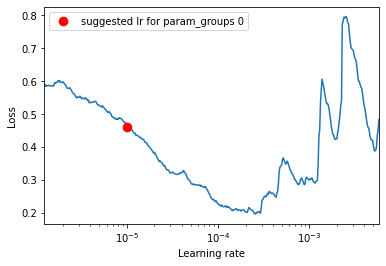# How to use FastaiLRFinder with Ignite

This how-to guide demonstrates how we can leverage the `FastaiLRFinder` handler to find an optimal learning rate to train our model on. We will compare the results produced with and without using the handler for better understanding.

In this example, we will be using a ResNet18 model on the MNIST dataset. The base code is the same as used in the Getting Started Guide.

## Basic Setup

``````import torch
import torch.nn as nn
from torch.utils.data import DataLoader
from torchvision.datasets import MNIST
from torchvision.models import resnet18
from torchvision.transforms import Compose, Normalize, ToTensor

from ignite.engine import create_supervised_trainer, create_supervised_evaluator
from ignite.metrics import Accuracy, Loss
from ignite.handlers import FastaiLRFinder
``````
``````device = torch.device("cuda" if torch.cuda.is_available() else "cpu")

class Net(nn.Module):
def __init__(self):
super(Net, self).__init__()

self.model = resnet18(num_classes=10)
self.model.conv1 = nn.Conv2d(
1, 64, kernel_size=3, padding=1, bias=False
)

def forward(self, x):
return self.model(x)

model = Net().to(device)

data_transform = Compose([ToTensor(), Normalize((0.1307,), (0.3081,))])

batch_size=128,
shuffle=True,
)

batch_size=256,
shuffle=False,
)

model = Net().to(device)
optimizer = torch.optim.RMSprop(model.parameters(), lr=1e-06)
criterion = nn.CrossEntropyLoss()
``````

We will first train the model with a fixed learning rate (lr) of 1e-06 and inspect our results. Let’s save the initial state of the model and the optimizer to restore them later for comparision.

``````init_model_state = model.state_dict()
init_opt_state = optimizer.state_dict()
``````

## Without LR Finder

``````trainer = create_supervised_trainer(model, optimizer, criterion, device=device)

``````
``````State:
iteration: 1407
epoch: 3
epoch_length: 469
max_epochs: 3
output: 0.5554001927375793
batch: <class 'list'>
metrics: <class 'dict'>
seed: <class 'NoneType'>
times: <class 'dict'>
``````
``````evaluator = create_supervised_evaluator(
model, metrics={"Accuracy": Accuracy(), "Loss": Loss(criterion)}, device=device
)

print(evaluator.state.metrics)
``````
``````{'Accuracy': 0.8655, 'Loss': 0.602867822265625}
``````

Let’s see how we can achieve better results by using the `FastaiLRFinder` handler. But first, let’s restore the initial state of the model and optimizer so we can re-train them from scratch.

``````model.load_state_dict(init_model_state)
``````

## With LR Finder

When attached to the `trainer`, this handler follows the same procedure used by fastai. The model is trained for `num_iter` iterations while the learning rate is increased from `start_lr` (defaults to initial value specified by the optimizer, here 1e-06) to the upper bound called `end_lr`. This increase can be linear (`step_mode="linear"`) or exponential (`step_mode="exp"`). The default `step_mode` is exponential which is recommended for larger learning rate ranges while linear provides good results for small ranges.

``````lr_finder = FastaiLRFinder()

# To restore the model's and optimizer's states after running the LR Finder
to_save = {"model": model, "optimizer": optimizer}

with lr_finder.attach(trainer, to_save, end_lr=1e-02) as trainer_with_lr_finder:
``````

Let’s plot how the learning rate changes within our specified range and print the suggested learning rate.

``````lr_finder.plot()

print("Suggested LR", lr_finder.lr_suggestion())
````````````Suggested LR 1.0148376909312998e-05
``````

Now we will apply the suggested learning rate to the optimizer, and train the model again with optimal learning rate.

``````lr_finder.apply_suggested_lr(optimizer)
print(optimizer.param_groups["lr"])
``````
``````1.0148376909312998e-05
``````
``````trainer.run(train_loader, max_epochs=3)
``````
``````State:
iteration: 1407
epoch: 3
epoch_length: 469
max_epochs: 3
output: 0.09644963592290878
batch: <class 'list'>
metrics: <class 'dict'>
``````# Calculate the new metrics after using the optimal lr
``````{'Accuracy': 0.9715, 'Loss': 0.0908882568359375}